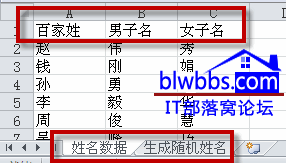# 利用excel中vba代码随机生成姓名列表的方法

[日期：2012-02-21]   来源：IT部落窝  作者：IT部落窝   阅读：4664[字体： ]

窝窝论坛上我们每天都会发布excel练习题给窝友们学习，在出题时，经常会遇到模拟随机生成姓名和数据。

数字可以使用rand、RANDBETWEEN函数来随机生成。可是姓名如何弄呢，本文介绍使用VBA自动随机生成姓名。

具体的随机生成姓名如下介绍：

第一步，新建一个excel工作薄

这个工作薄里面包含两个工作表，分别是“姓名数据”和“生成随机姓名”。

其中的“姓名数据”工作表，有三列数据：百家姓、男子名、女子名，是我们之前已经输入好了的。第二步，切换到“生成随机姓名”工作表，单击“生成随机姓名”按钮，excel会出现一个输入框，提示我们输入要生成的人名数。比如我们输入100，单击“确定”，此工作表A列就会随机生成姓名100个。下面提供随机生成姓名的VBA代码，使用方法是按下ALT+F11组合键，打开VBE编辑器，插入——模块，复制下面的代码，然后为了操作方便，我们在“生成随机姓名”工作表中插入了一个按钮，将这段代码指定给按钮。

Sub 随机生成姓名()
Dim Arr, Arr2, Arr3, I%, N&, S As Boolean, Str\$, Dic, A&, B&
N = Val(InputBox("请输入要生成的人名数："))     '输入要生成的随机人名数量
If N = 0 Then Exit Sub      '如果未输入或点了取消则退出程序
Set Dic = CreateObject("scripting.dictionary")  '创建字典项目，用于清除生成的重复的人名
With Sheet1     '从sheet1中读取数据并随机生成姓名
Arr = .Range(.[a2], .Cells(.Rows.Count, 1).End(3))  '读取百家姓放入数组
Arr2 = .Range(.[b2], .Cells(.Rows.Count, 2).End(3))    '读取男子名常用字放入数组
Arr3 = .Range(.[c2], .Cells(.Rows.Count, 3).End(3))     '读取女子名常用字放入数组
Do  '循环执行
Str = ""       '先清空用于存储生成的名字的变量
S = Rnd > 0.5   '用取得的随机数来判断生成男子名还是女子名
I = 2 + IIf(Rnd > 0.8, 0, 1)    '用随机数确定生成的名字的字符数（80%的几率为三个字）
If S Then   '如果是男子名，则
A = Int(Rnd * (UBound(Arr) - 2)) + 2    '取得姓对应的数组下标
B = Int(Rnd * (UBound(Arr2) - 2)) + 2   '取得名字的对应下数组下标
Str = Arr(A, 1) & Arr2(B, 1)    '串接名字
If I > 2 Then   '如果名字的字符数大于2，则再得一次名字的字符下标并串接给字符串变量
B = Int(Rnd * (UBound(Arr2) - 2)) + 2
Str = Str & Arr2(B, 1)
End If
Else    '如果是女子名，则取对应的女子名数组内容，处理方式同上
A = Int(Rnd * (UBound(Arr) - 2)) + 2
B = Int(Rnd * (UBound(Arr3) - 2)) + 2
Str = Arr(A, 1) & Arr3(B, 1)
If I > 2 Then
B = Int(Rnd * (UBound(Arr3) - 2)) + 2
Str = Str & Arr3(B, 1)
End If
End If
Dic(Str) = ""   '将随机姓名放入字典中
Loop Until Dic.Count = N    '如果字典项目数量达到输入的数值则跳出循环
End With
With Sheet2     '将结果写入sheet2中
.Cells.Clear    '清空原有内容
If Dic.Count > 65536 Then   '防止数量太多时无法转置及数据超出单元格最大行号的情况
Arr = Dic.keys
For N = LBound(Arr) To UBound(Arr)
Cells((N Mod 65536) + 1, N \ 65536 + 1) = Arr(N)
Next N
Else    '数量少时直接转置
.[a1].Resize(Dic.Count, 1) = Application.Transpose(Dic.keys)    '转置字典的keys值
End If
End With
Set Dic = Nothing   '清空字典项目
End Sub分享到： QQ空间 新浪微博 腾讯微博 人人网